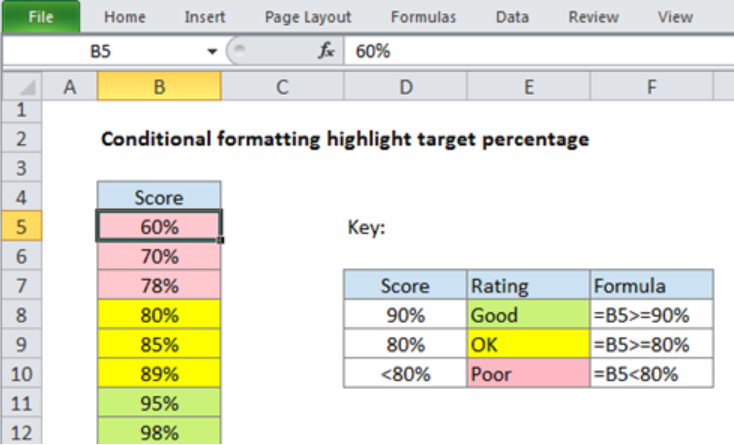Get instant live expert help with Excel or Google Sheets“My Excelchat expert helped me in less than 20 minutes, saving me what would have been 5 hours of work!”

#### Post your problem and you’ll get expert help in seconds.

Your message must be at least 40 characters
Our professional experts are available now. Your privacy is guaranteed.

# Conditional formatting highlight target percentage

We can use multiple conditional formatting rules to highlight percentage values using different colors. With multiple conditional formatting, we can different rules each with its own threshold. This article provides a clear guide on how to use conditional formatting highlight target percentage.Figure 1: Conditional formatting to highlight target percentage

## General syntax of the formula

`=A1>= X`

`=A1>= Y`

`=A1< Y`

## Understanding the formula

The formula above is fundamental when we want to highlight percentages values of different cells using different colors. We need to apply conditional formatting for us to get the various colors we want.

## How the formula works

In figure 1 above, we have formatted the cells with the following colors;

`=B5>=90% –Green`

`=B5>=80% -Yellow`

`=B580% -Pink`

• The first formula is first evaluated against each cell in the specified range B5:B12. Here, the formula will return TRUE for all those cells whose values are greater or equal to 90%. It will return a FALSE if the condition is not met.
• The second formula is also evaluated against all the cells in range B5:B12. This formula returns TRUE if the values are greater or equals to 80%, and FALSE if the not. The cells that meet this condition are then filled with yellow.
• The third formula is then evaluated against the cells in the range B5:B12 to check whether the values are less than 80%. If the condition is met, the formula shall return TRUE and the cells whose values are less than 80% are filled with pink.

Note that for the first two rules, we need to enable the “stop if true” option. This is to prevent further processing given that the rules are mutually exclusive.

## Instant Connection to an Expert through our Excelchat Service

Most of the time, the problem you will need to solve will be more complex than a simple application of a formula or function. If you want to save hours of research and frustration, try our live Excelchat service! Our Excel Experts are available 24/7 to answer any Excel question you may have. We guarantee a connection within 30 seconds and a customized solution within 20 minutes.

Are you still looking for help with Conditional Formatting? View our comprehensive round-up of Conditional Formatting tutorials here.

### Did this post not answer your question? Get a solution from connecting with the expert.Another blog reader asked this question today on Excelchat:
Solution examplesUse the Vlookup Function to complete the "employee" column of table 2. Use "job Id" from table 2 as your lookup_value(s) and table 1 as your reference.
Solved by C. H. in 16 minsIf a cell in another sheet is populated I need a vlookup done. If the cell is not populated I need the cell to return blank.
Solved by T. D. in 60 minsI am trying to make a chart that turns a week range red if nothing is entered in the range. If something is entered then I would like it to turn green. Please Help
Solved by E. U. in 43 minsI need a check box to show/hide an answer of an if function
Solved by Z. U. in 23 minsI need a formula to compare the data in two columns and then export the mismatched data in the 3rd column
Solved by S. Q. in 20 mins## Subscribe to Excelchat.coAnother blog reader asked this question today on Excelchat: